Click to Chat

1800-1023-196

+91-120-4616500

CART 0

• 0

MY CART (5)

Use Coupon: CART20 and get 20% off on all online Study Material

ITEM
DETAILS
MRP
DISCOUNT
FINAL PRICE
Total Price: Rs.

There are no items in this cart.
Continue Shopping• Complete Physics Course - Class 11
• OFFERED PRICE: Rs. 2,968
• View Details

```Total Internal Reflection

Table of Content

Introduction to Total Internal Reflection

What is Critical Angle?

Conditions for Total Internal Reflection

Facts of Total Internal Reflection

Applications of Total Internal Reflection

Conclusion

Introduction to Total Internal Reflection

Total Internal Reflection is a scenario where a travelling wave of light strikes a boundary of a medium but at an angle that is larger than critical angle. So, the concept behind is that if the refractive index on the other side of the boundary of the medium is lower, and the light wave strikes at greater angle, so the wave is reflected internally entirely. Total internal reflection is a basic concept used in variety of applications for fast travelling of light rays. Total Internal Reflection can also be extended onto various concepts that are arose out of it like frustrated total internal reflection or evanescent waves which are the special cases of this phenomena.

The following discussion will ponder over the reasons for total internal reflection, the scenarios where it occurs, some special cases of total internal reflection and finally some great applications of total internal reflection in our day to day lives. The following figure gives a day to day observation of total internal reflection in dark when a laser beam reflects internally and traverses across the glass tube. See Figure 1 below.Figure 1: Example of Total Internal Reflection

What is Critical Angle?

Critical Angle is a specific angle where if the light travels from denser to rarer medium at this angle it goes in a straight line across the boundary. To understand better this phenomenon, we use ray diagram. In the figure 2 shown, a ray of light AO is travelling from denser to rarer medium with small incidence angle i.e. when the ray reaches into rarer medium it bend away from normal ON and refracts with an angle r in the rarer medium and goes in direction OC.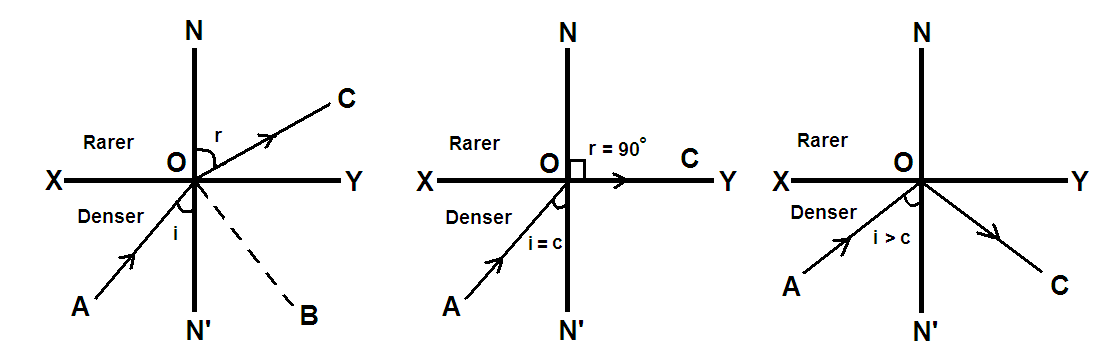Figure 2: Ray diagram to explain critical angle

When ray of light bends away from the normal in rarer region, the angle of reflection in rarer medium is greater than the angle of incidence i in denser medium.

By increasing the angle of incidence in denser medium, the angle of refraction r in denser region increases gradually. For a certain angle of incidence angle i = c, the angle of refraction in rarer medium becomes 90° and thus known as critical angle.

We should know the critical angle of glass, which is 42°, which means when we will incident a ray at an angle of 42°, and then only we will get angle of refraction in air as 90°.

The critical angle for a medium is thus defined as the angle of incidence in medium for which refraction angle in air is 90°. The critical angle is donated by letter c.

Conditions for Total Internal Reflection

For an angle of incidence greater than critical angle c, there will be no refraction of light from denser to rarer medium which is usually the case when light travels so on angles lesser than the critical angle. That means all light comes back, that means it is reflected back in the denser medium and is shown in diagram 3 of Figure 2 with ray OC. Here, no refraction is present at all. All the light is reflected back into the denser medium. This is the underlying concept of total internal reflection where instead of refraction an internal reflection takes place.

Angle of incidence when travelling from denser medium is more than the critical angle of the medium, then no refraction occurs in the rarer medium, all the light gets reflected back into the denser medium. We define it as total internal reflection. The conditions that are necessary and mandatory for the phenomena of total internal reflection are:

Light should pass from denser to rarer medium (in the terms of refractive index of a material)

Angle of incidence formed in denser medium should be more than critical angle of the medium (if less, then the light will be refracted).

Snell’s law does not apply here as there is no refraction and thus follow law of reflection.

Total internal reflection will happen when the light will travel from water towards air but the scenario when it travels from air towards water will not occur. This is one of the examples and it will be same in case of glass to air, glass or glass to water and any two media of different refractive indices.

The case to ponder is that how big the angle of incidence be? For example, the critical angle for water-air interface is 48.6 so any angle of incidence which is even slightly greater than this critical angle will result in total internal reflection. The comparison only lies in exceeding the critical angle and not in the amount by which it is exceeded. On every angle that is greater than the critical angle, total internal reflection will definitely occur regardless of the shape of the media itself. Critical angle depends upon the media selected for the travelling of light rays.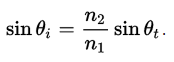So, Ɵi (angle of incidence) is calculated by keeping Ɵt as 90 degrees. Here, n2 and n1 both are the refractive indices of the media.

Since, critical angle is media dependent, so for every pair of media there are different critical angles and hence different angle of incidence greater than corresponding critical angles will result in total internal reflection phenomena.Figure 3: Scenario of Total Internal Reflection

Facts of Total Internal Reflection

The total internally reflected light beam has a phase shift between incident and reflected light ray which is dependent upon the angle. This phase shift which is occurring is polarization dependent. This is always the case in total internal reflection. A π phase shift occurs at an angle known as Brewster’s angle which indicates a changes in refection coefficient when it passes through zero. As the angle of incidence deviates more from the critical angle and near to the grazing incidence, this phase shift grows as a result. This has application in the design of Fresnel rhomb which is used to convert the circular polarization into linear polarization or the other way round for different colors (wavelengths).

Another concept of total internal reflection is known as frustrated total internal reflection according to which, if a third medium having a higher refractive index is placed within a specific wavelength distance from the interface of first and second medium, the evanescent waves will pass energy from second to third medium, leading to the phenomena similar to quantum tunnelling. This concept is also used in many of the applications of total internal reflection. The following figure 4 illustrates the concept of frustrated total internal reflection in the case of glass prisms and air.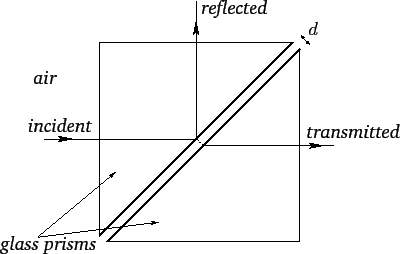Figure 4: Frustrated total internal reflection

One of the disadvantages that come along total internal reflection is production of evanescent waves which are beyond the surface of the boundary. This is caused due to the fact that some of the light gets penetrated into the second medium on the point of their boundary. Hence, this wave of light tends to travel alongside the boundary that lies between the two materials. This can be a rare chance but has applications too.

Applications of Total Internal Reflection

Total Internal Reflection is primarily used in the field of fibre optics. The structure of the optical fibre makes the total internal reflection possible. There is a difference in refractive index of core and cladding which makes it possible for the ray of the light to reflect internally. By using this technique, the light remains trapped into the optic fibre cable and this phenomenon helps in fast transfer of light down the entire length. Optic fibres are mainly used in the field of telecommunications wherein there is a requirement of making it to travel through long distances without getting lost or distorted. Hence, in this light will always be reflecting from the layer of cladding having lower refractive index regardless of the angle that the fibre bends into i.e. the shape of the fibre cable which may even take the shape of a circle.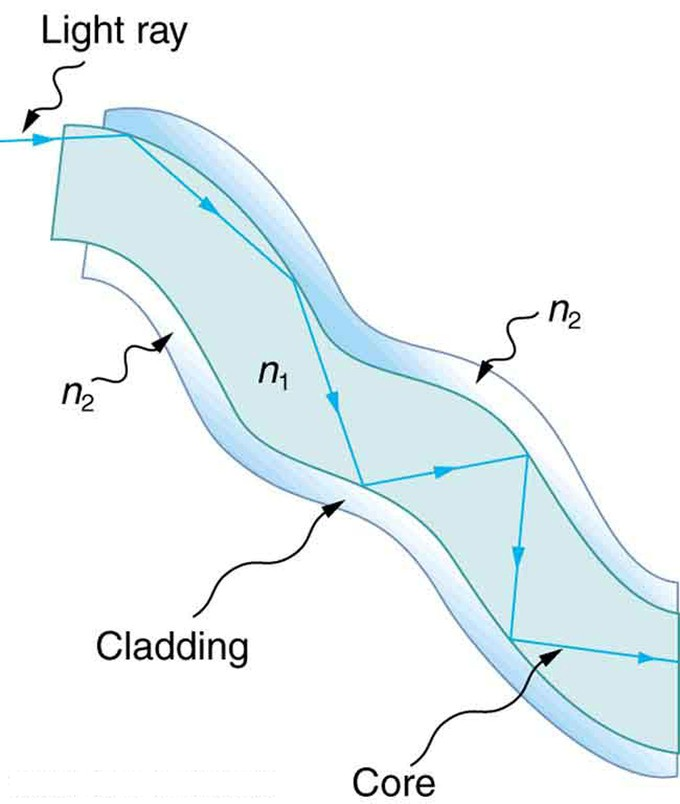Figure 5: Total Internal Reflection in Fibre Optics

Another application of total internal reflection is in medical science where this concept is applied to endoscopic tests where there is a need for the light to reflect internally. Prisms used in binoculars uses this concept to show erect images. Optical fingerprinting is another application used to record the image of the fingerprint. LED light panels are also based on the principle of total internal reflection.

Diamonds partly get their brilliance and sparkle from the concept of this total internal reflection. This is because of the high refractive index of the diamond which is 2.3. so, the diamond achieves it’s shine when the light ray strikes many internal surfaces of diamond (due to it’s cut) and then at one particular incident when the angle is less that critical angle of 25 degrees, it escapes from the medium and emerges into the air. This is the cause when the colours of the light gets separated and are shown brilliantly.

Some other major applications of total internal reflection include controller of the automatic windshield wipers in the case of rain sensors, used in spatial light filtering, used in prisms that are used in binoculars in order to show the images erect. These are very useful in the recent technology wherein multi-touch screens are used which use the concept of frustrated total internal reflection to sense different targets at the same time. A fluorescence microscope that uses this principle based on evanescent waves excite the material fluorophores to closely monitor and observe biological samples. Prisms can also be modified in order to observe total internal reflection.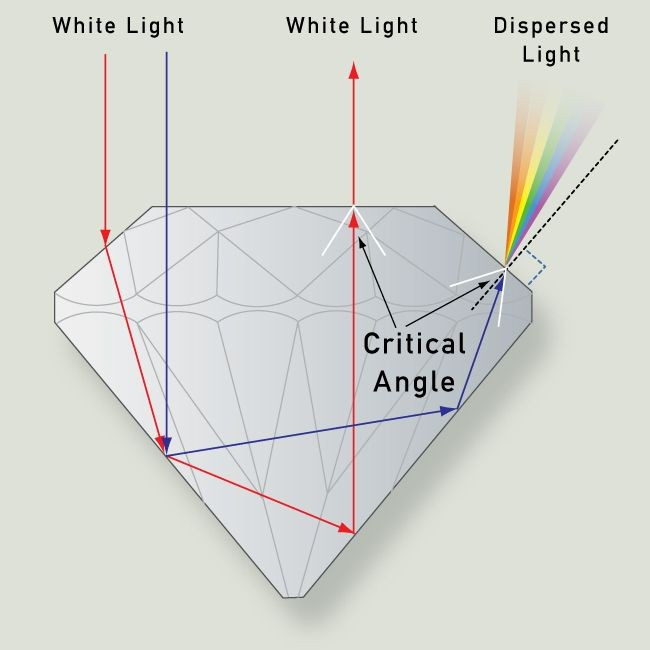Figure 6: Total Internal Reflection in diamond

Conclusion

Total Internal Reflection is a phenomena which is involved in various practices of our lives unknowingly and is cause of various applications in our everyday lives. It simple occurs because of the difference in the refractive indices of two mediums which are bundled together and hence, light traps in between them due to some specific angle of incidents (greater than critical angle). Therefore, it is really important to have a deep idea about total internal reflection in order to understand the underlying principles of various things such optical fibres, endoscopy and variety of routine things too. Total internal reflection hence forms basis of various other phenomena that help in medical science, telecommunication etc.

Total Internal Reflection
```### Course Features

• 101 Video Lectures
• Revision Notes
• Previous Year Papers
• Mind Map
• Study Planner
• NCERT Solutions
• Discussion Forum
• Test paper with Video Solution